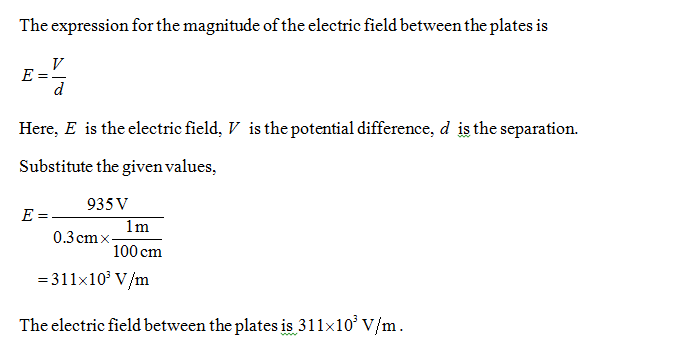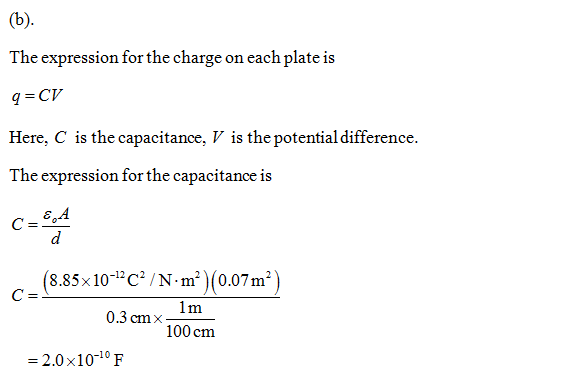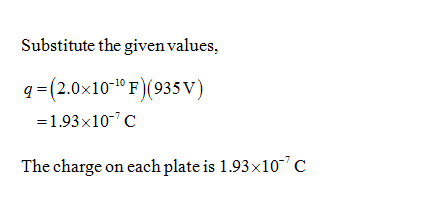# The plates of a parallel-plate capacitor are 700 cm2 in area and 0.3 cm apart. THe potential difference between the plates is 935 V. (a) What is the magnitude of the electruc field between the plates?(b) The charge on each plate?Now the two plates touch at the bottom forming a V-shape. (c) Find the total electric energy stored between the plates.

Question
7 views

The plates of a parallel-plate capacitor are 700 cmin area and 0.3 cm apart. THe potential difference between the plates is 935 V.

(a) What is the magnitude of the electruc field between the plates?

(b) The charge on each plate?

Now the two plates touch at the bottom forming a V-shape.

(c) Find the total electric energy stored between the plates.

check_circle

Step 1Step 2...

### Want to see the full answer?

See Solution

#### Want to see this answer and more?

Solutions are written by subject experts who are available 24/7. Questions are typically answered within 1 hour.*

See Solution
*Response times may vary by subject and question.
Tagged in